# A 100.-mL reaction vessel initially contains 2.60 × 10–2 mol of NO and 1.30 × 10–2 mol of H2. At equilibrium, the concentration of NO in the vessel is 0.161 M. At equilibrium the vessel also contains N2, H2O, and H2.The balanced equation for the reaction is given below. 2H2​(g)+2NO(g)<------>2H2​O(g)+N2(g)Determine the equilibrium concentration of N2.

Question
56 views

A 100.-mL reaction vessel initially contains 2.60 × 10–2 mol of NO and 1.30 × 10–2 mol of H2. At equilibrium, the concentration of NO in the vessel is 0.161 M. At equilibrium the vessel also contains N2, H2O, and H2.

The balanced equation for the reaction is given below.

2H2​(g)+2NO(g)<------>2H2​O(g)+N2(g)

Determine the equilibrium concentration of N2.

check_circle

Step 1

Given information with unit conversion: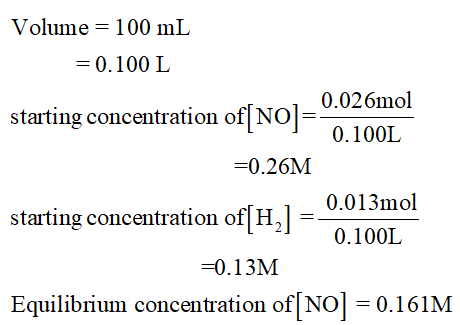Step 2

The balanced equation can be written as,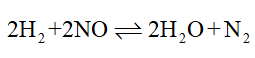The equilibrium concentration of N2 can be determined as,

ICE table: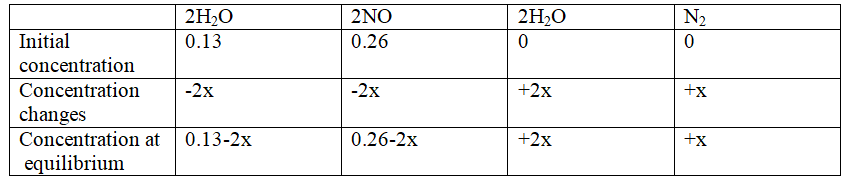Step 3

The value of x is concentration changes in the chemical process

The concentration changes can be calculated (At equilibrium)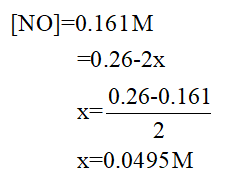The concentration of H2 at equilibrium,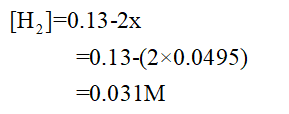...

### Want to see the full answer?

See Solution

#### Want to see this answer and more?

Solutions are written by subject experts who are available 24/7. Questions are typically answered within 1 hour.*

See Solution
*Response times may vary by subject and question.
Tagged in